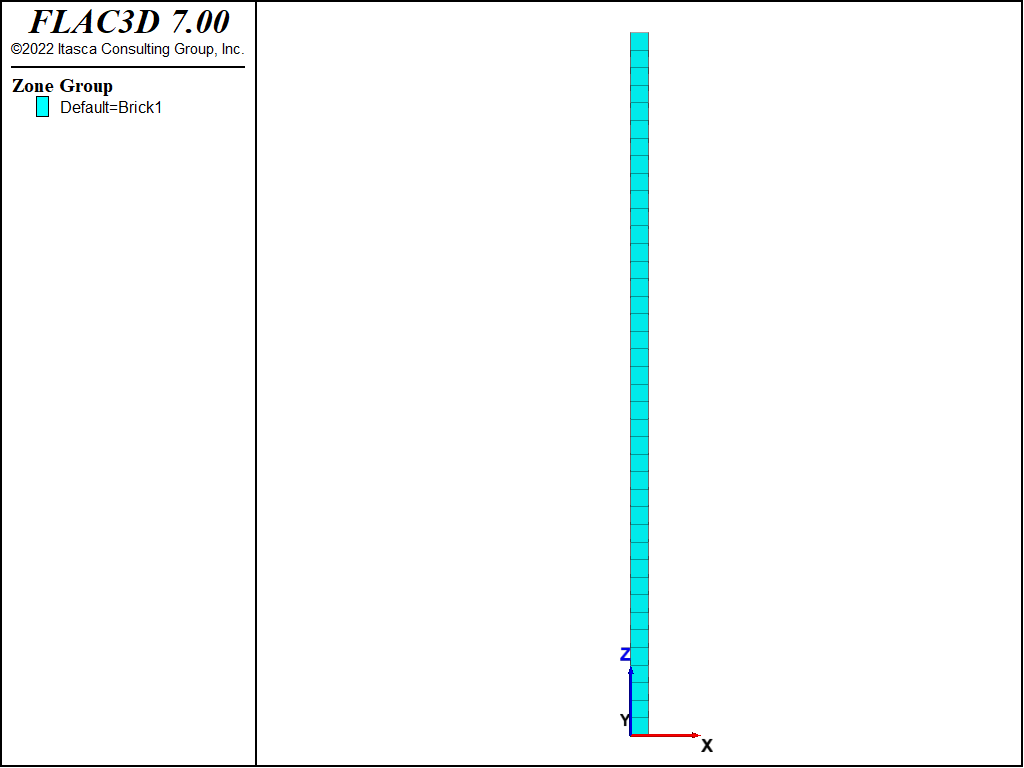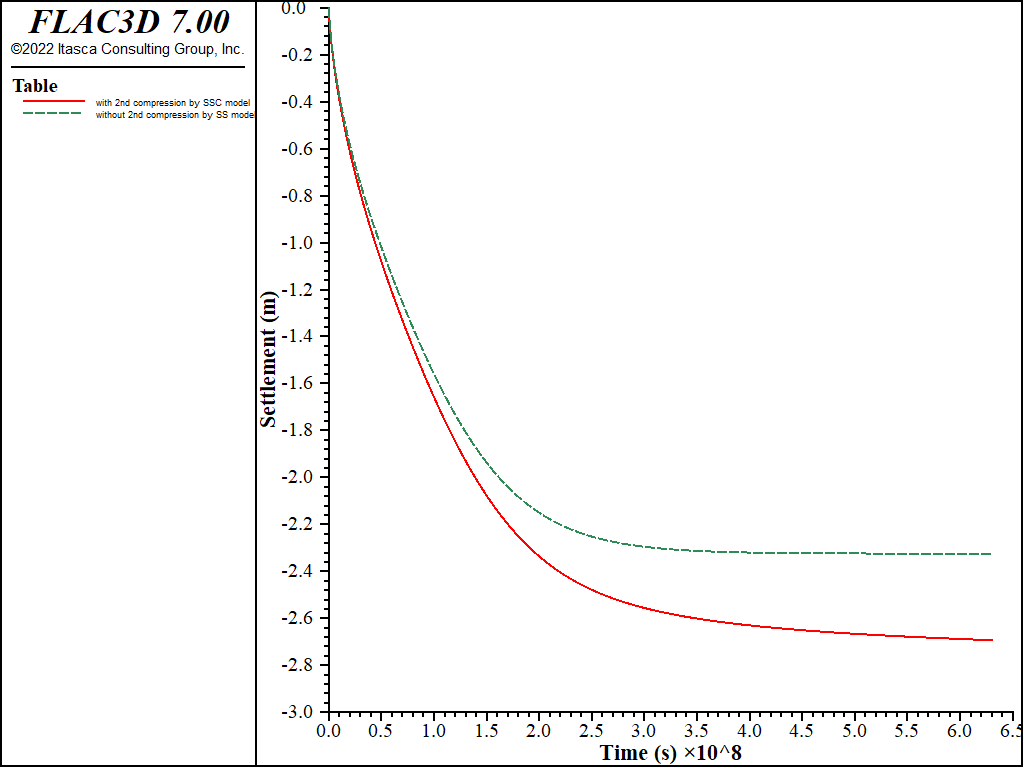Examples • Example Applications

# One-Dimensional Consolidation Considering Secondary Compression

Note

The project file for this example is available to be viewed/run in FLAC3D. The project’s main data files are shown at the end of this example.

A saturated layer of soil of thickness $$H$$ = 10 m and large horizontal extent rests on a rigid impervious base. A constant surface load, $$p_z$$ = 4 × 105 Pa, is applied on the layer under undrained conditions. The soil matrix is homogeneous soft soil and modeled by Soft-Soil-Creep (SSC) and by Soft-Soil (SS) to check the secondary compression effect; a uniform effective vertical stress $$\sigma'_{v0}$$ = 4 × 104 Pa is assumed for the soil layer for simplicity; the isotropic Darcy’s transport law applies. The applied pressure is initially carried by the fluid, but as time goes on the fluid drains through the layer surface, transferring the load to the soil matrix. The drainage is two-way.

The following parameters are prescribed for this example:

 specific density of soil solid, $$G_s$$ 2.78 initial void ratio, $$e_0$$ 2.7 permeability (conventional), $$k$$ 5 × 10-10 m/s $$C_c$$ 1.0 $$C_s$$ 0.1 friction at critical state 30 degrees Poisson’s ratio 0.2 OCR 1.0 $$K_{nc}$$ 0.5 water bulk modulus, $$K_w$$ 2 × 108 Pa

For SSC model, two additional parameters are: reference time $$\tau$$ = 2200 seconds and $$C_\alpha$$ = 0.04. Two scenarios are analyzed - A: considering secondary compression (creep), realized by using SSC model to the soil matrix; and B: not considering the secondary compression (creep), realized by using SS model to the soil matrix.

The FLAC3D model grid for this problem is a column of 40 zones of unit dimensions lined up along the $$z$$-axis. (See Figure 1.) The base of the column is fixed, and lateral displacements are restricted in the $$x$$- and $$y$$-directions. A mechanical pressure, $$p_z$$, is applied at the top of the column.

At first, flow is prevented and the model is stepped to equilibrium to establish the initial undrained conditions. At the end of this stage, the stress $${\sigma}_{zz}$$ has the value $$-(p_z+\sigma'_{v0})$$, in equilibrium with the applied pressure. Drainage is then allowed by setting the pore pressure to zero at the top and base of the column. The FLAC3D model is cycled to a flow time of 20 years.

breakFigure 1: FLAC3D grid for one-dimensional consolidation.Figure 2: Comparison of excess pore pressure with and without secondary compression (creep) at various times in a 1D consolidation test.Figure 3: Comparison of vertical displacement with and without secondary compression (creep) in a 1D consolidation test.

Figure 2 presents the excess pore pressure (EPP) at time of 0.5. 1, 2,, 3, 4, 5, 6, and 20 years for scenario A and B. At the same time, this example shows quicker EPP dissipation if considering the secondary compression.

Figure 3 plots the vertical displacement (settlement) for 20 years. It is clear that the settlement is starting flatted to about 2.2 m from about 3.5 years if not considering the secondary compression, while the settlement is continuously increasing if considering the secondary compression.

Data File

1DConsolidation-Coupled.dat

model new
model title "One-dimensional consolidation"
model large-strain off
fish automatic-create off
model configure fluid
;
program call "parameters.dat"
[parameters]
;
zone create brick size 1 1 [nz] point 0 (0,0,0) point 1 ([maxx],0,0) ...
point 2 (0,[maxy],0) point 3 (0,0,[maxz])
zone face skin
zone cmodel assign soft-soil
zone property density [rhod] lambda-modified [lambda] kappa-modified [kappa]
zone property poisson [pr] friction [fri] o-c-r [ocr] c-n-c [knc]
zone property void-initial [e0]
zone property stress-1-eff [-sv0] stress-2-eff [-knc*sv0] stress-3-eff [-knc*sv0]
zone face apply velocity-x 0.0 range group 'West' or 'East'
zone face apply velocity-y 0.0 range group 'North' or 'South'
zone face apply velocity-z 0.0 range group 'Bottom'
zone initialize stress-xx [-knc*sv0]
zone initialize stress-yy [-knc*sv0]
zone initialize stress-zz [-sv0]
; --- fluid flow model ---
zone fluid cmodel assign isotropic
zone fluid property permeability [perm] porosity [poro]
zone gridpoint initialize saturation 1.0
zone gridpoint initialize fluid-modulus 2e8
zone gridpoint initialize pore-pressure 0
zone initialize fluid-density [rhow]
;
[global gpdiz = gp.near(maxx,maxy,maxz)]
;
model fluid active off
zone face apply stress-zz [-sv0-svd] servo ramp minimum [1.0/11] range group 'Top'
model solve ratio 1e-7
;
zone gridpoint initialize disp (0,0,0)
; variable records
fish define ft
global ft = fluid.time.total
global gpdztop = gp.disp.z(gpdiz)
end
; outputs
[global pparray = array.create(8,nz+1)]
fish define get_pp(j)
zone.field.name = 'pore-pressure'
zone.field.method.name = 'poly'
loop local kk (1,nz+1)
pparray(j,kk) = zone.field.get(0,0,hh*float(kk-1)/float(nz))
endloop
end
; --- histories ---
history interval 1000
fish history name 'time'  ft
fish history name 'dztop' gpdztop
;
model fluid active on
model fluid time-total 0.0
;
zone face apply pore-pressure 0 range group 'Top'
zone face apply pore-pressure 0 range group 'Bottom'
model fluid active on
model fluid substep 1
model mech substep 1
model mech slave on
;
model solve time-total [0.5*365.0*24.0*3600.0]
[get_pp(1)]
model solve time-total [1.0*365.0*24.0*3600.0]
[get_pp(2)]
model solve time-total [2.0*365.0*24.0*3600.0]
[get_pp(3)]
model solve time-total [3.0*365.0*24.0*3600.0]
[get_pp(4)]
model solve time-total [4.0*365.0*24.0*3600.0]
[get_pp(5)]
model solve time-total [5.0*365.0*24.0*3600.0]
[get_pp(6)]
model solve time-total [6.0*365.0*24.0*3600.0]
[get_pp(7)]
model solve time-total [20.0*365.0*24.0*3600.0]
[get_pp(8)]
;
history export 'dztop' vs 'time' table 'settlement_nocreep'
table 'settlement_nocreep' export 'settlement_nocreep' truncate
;
[io('pp_nocreep.csv',pparray)]
model save 'noCreep'


1DConsolidation-Coupled10M.dat

model new
model title "One-dimensional consolidation with Soft-Soil-Creep model"
model large-strain off
fish automatic-create off
model configure fluid creep
;
program call "parameters.dat"
[parameters]
;
zone create brick size 1 1 [nz] point 0 (0,0,0) point 1 ([maxx],0,0) ...
point 2 (0,[maxy],0) point 3 (0,0,[maxz])
zone face skin
zone cmodel assign soft-soil-creep
zone property density [rhod] lambda-modified [lambda] kappa-modified [kappa]
zone property poisson [pr] friction [fri] o-c-r [ocr] c-n-c [knc]
zone property index-creep [mu] time-reference 2200
zone property void-initial [e0]
zone property stress-1-eff [-sv0] stress-2-eff [-knc*sv0] stress-3-eff [-knc*sv0]
zone face apply velocity-x 0.0 range group 'West' or 'East'
zone face apply velocity-y 0.0 range group 'North' or 'South'
zone face apply velocity-z 0.0 range group 'Bottom'
zone initialize stress-xx [-knc*sv0]
zone initialize stress-yy [-knc*sv0]
zone initialize stress-zz [-sv0]
; --- fluid flow model ---
zone fluid cmodel assign isotropic
zone fluid property permeability [perm] porosity [poro]
zone gridpoint initialize saturation 1.0
zone gridpoint initialize fluid-modulus 2e8
zone gridpoint initialize pore-pressure 0
zone initialize fluid-density [rhow]
;
[global gpdiz = gp.near(maxx,maxy,maxz)]
;
model fluid active off
model creep active off
zone face apply stress-zz [-sv0-svd] servo ramp minimum [1.0/11] range group 'Top'
model solve ratio 1e-7
;
zone gridpoint initialize disp (0,0,0)
; variable records
fish define ft
global ft = fluid.time.total
global gpdztop = gp.disp.z(gpdiz)
end
; outputs
[global pparray = array.create(8,nz+1)]
fish define get_pp(j)
zone.field.name = 'pore-pressure'
zone.field.method.name = 'poly'
loop local kk (1,nz+1)
pparray(j,kk) = zone.field.get(0,0,hh*float(kk-1)/float(nz))
endloop
end
; --- histories ---
history interval 1000
fish history name 'time'  ft
fish history name 'dztop' gpdztop
;
model fluid active on
model fluid time-total 0.0
;
model creep active on
model creep time-total 0.0
model creep timestep starting 1.e-4 minimum  1.e-4 maximum  20000.0 latency 10
;
zone face apply pore-pressure 0 range group 'Top'
zone face apply pore-pressure 0 range group 'Bottom'
;
model solve time-total [0.5*365.0*24.0*3600.0]
[get_pp(1)]
model solve time-total [1.0*365.0*24.0*3600.0]
[get_pp(2)]
model solve time-total [2.0*365.0*24.0*3600.0]
[get_pp(3)]
model solve time-total [3.0*365.0*24.0*3600.0]
[get_pp(4)]
model solve time-total [4.0*365.0*24.0*3600.0]
[get_pp(5)]
model solve time-total [5.0*365.0*24.0*3600.0]
[get_pp(6)]
model solve time-total [6.0*365.0*24.0*3600.0]
[get_pp(7)]
model solve time-total [20.0*365.0*24.0*3600.0]
[get_pp(8)]
;
history export 'dztop' vs 'time' table 'settlement_withcreep'
table 'settlement_withcreep' export 'settlement_withcreep' truncate
;
[io('pp_withcreep.csv',pparray)]
model save 'withCreep'


Endnotes

  To view this project in FLAC3D, use the program menu. Help ▼ Examples…   ⮡   FLAC     ⮡   Example Applications       ⮡   1dConsolidationSecondaryCompression         ⮡   1dConsolidationSecondaryCompression.prj